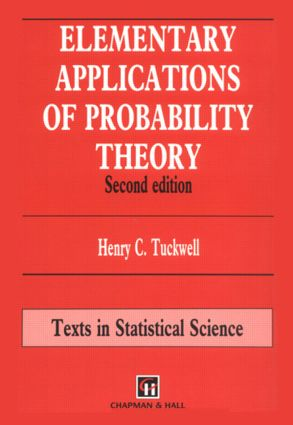Elementary Applications of Probability Theory

2nd Edition

Chapman and Hall/CRC

308 pages

Purchasing Options:\$ = USD
Hardback: 9780412576201
pub: 1995-05-15
SAVE ~\$19.19
\$95.95
\$76.76
x
eBook (VitalSource) : 9780203758564
pub: 2018-02-06
from \$28.98

FREE Standard Shipping!

Description

This book provides a clear and straightforward introduction to applications of probability theory with examples given in the biological sciences and engineering.

The first chapter contains a summary of basic probability theory. Chapters two to five deal with random variables and their applications. Topics covered include geometric probability, estimation of animal and plant populations, reliability theory and computer simulation. Chapter six contains a lucid account of the convergence of sequences of random variables, with emphasis on the central limit theorem and the weak law of numbers. The next four chapters introduce random processes, including random walks and Markov chains illustrated by examples in population genetics and population growth.

This edition also includes two chapters which introduce, in a manifestly readable fashion, the topic of stochastic differential equations and their applications.

Preface

A Review of Basic Probability Theory

Geometric Probability

Some Applications of the Hypergeometric and Poisson Distributions

Reliability Theory

Simulation and Random Numbers

Convergence of Sequences of Random Variables: The Central Limit Theorem and the Laws of Large Numbers

Simple Random Walks

Population Genetics and Markov Chains

Population Growth I: Birth and Death Processes

Population Growth II: Branching Processes

Stochastic Processes and an Introduction to Stochastic Differential Equations

Diffusion Processes, Stochastic Differential Equations and Applications

Appendix: Table of Critical Values of the X2-Distribution

Index